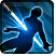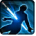# [alt_special_attack]Conditions

## Related effects, buffs and debuffs

Please click on an effect below to view its details.

• [does not expire][alt_special_attack]

 Slot: Debuff Duration: passive Tick rate: does not tick # occurrences: 0 Target overrides: [target override] Aoe Sphere
• On Apply

Perform the following actions:

• Play appearance epp . test . ammer . 250, dependent on calling effect
• When channel ended

Perform the following actions:

• Custom
- Param 2 = (string) ended
- Param 3 = (string)
- Param 4 = (string)
- Param 5 = (string)
- Param 6 = (string)
- Param 7 = (string)
- Param 8 = (string)
- Param 9 = (string)
- Param 1 = (int) 9499481598613081908
• [does not expire][alt_special_attack]

 Slot: Debuff Duration: passive Tick rate: does not tick # occurrences: 0
• When channel ended

Perform the following actions:

• Custom
- Param 2 = (string) ended
- Param 3 = (string)
- Param 4 = (string)
- Param 5 = (string)
- Param 6 = (string)
- Param 7 = (string)
- Param 8 = (string)
- Param 9 = (string)
- Param 1 = (int) 9499481598613081908
• When channel finished

Perform the following actions:

• Add effect #2 to TARGET from TARGET
• Custom
- Param 2 = (string) ended
- Param 3 = (string)
- Param 4 = (string)
- Param 5 = (string)
- Param 6 = (string)
- Param 7 = (string)
- Param 8 = (string)
- Param 9 = (string)
- Param 1 = (int) 9499481598613081908